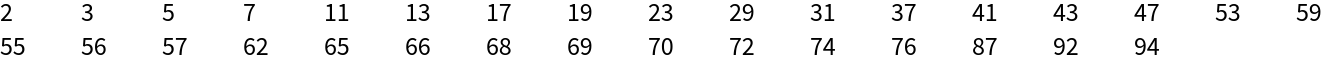Function Repository Resource:

# NestWhileGraph

Iteratively construct graphs up to a termination condition

 ResourceFunction["NestWhileGraph"][f,expr,test] generates a directed graph whose nodes are obtained by applying f repeatedly, starting from expr, and continuing until applying test to the result no longer yields True. ResourceFunction["NestWhileGraph"][f,expr,test,m] supplies a list of the most recent m results to test at each step. ResourceFunction["NestWhileGraph"][f,expr,test,All] supplies a list of all results so far to test at each step. ResourceFunction["NestWhileGraph"][f,expr,test,m,max] applies f at most max times.

## Details

ResourceFunction["NestWhileGraph"] accepts all the options of Graph.
When testing on more than one successive graph, results are time ordered with older results occurring earlier in the list.
Setting DirectedEdges to False returns an undirected graph.
Typically the iterator f should be a list-valued function whose elements are also valid inputs to f.
The most general form for a typical output allows internal cycles.
If an iteration returns to a previously visited value, it does not use that value as an input at the next step.
Output graphs will not have duplicate edges.

## Examples

### Basic Examples (3)

Construct a graph by applying f while VertexCount is less than 5:

 In:=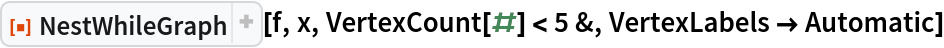Out=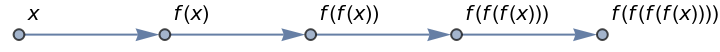Apply a similar termination condition only to growth-front subgraph:

 In:=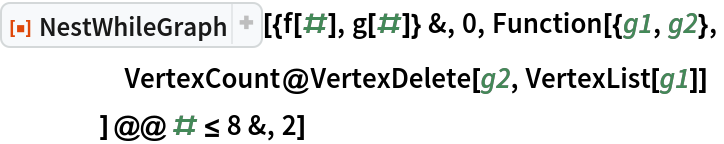Out=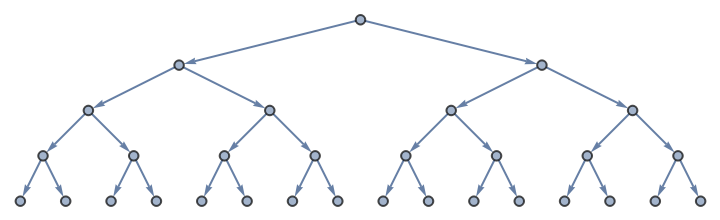Terminate the growth of a graph according to properties of its vertex set:

 In:=Out=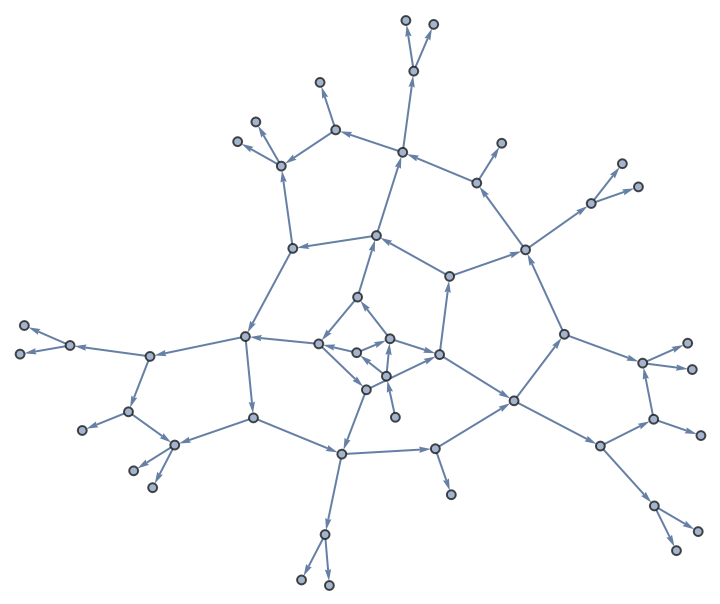Explore a grid graph through multiway spacetime evolution until every vertex has been visited:

 In:=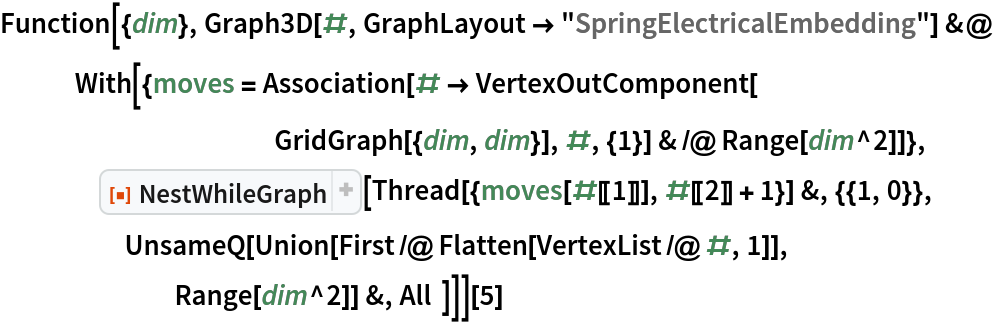Out=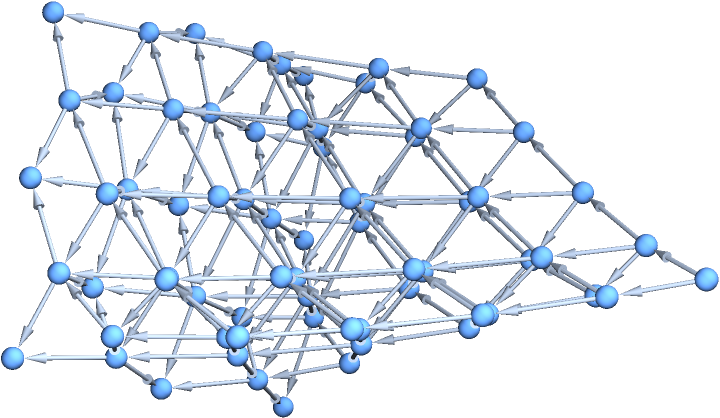Generate a torus graph:

 In:=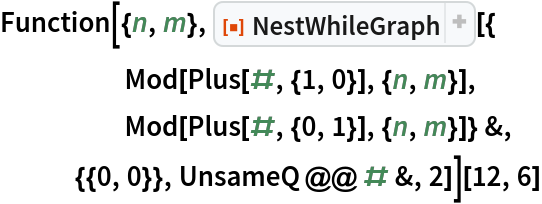Out=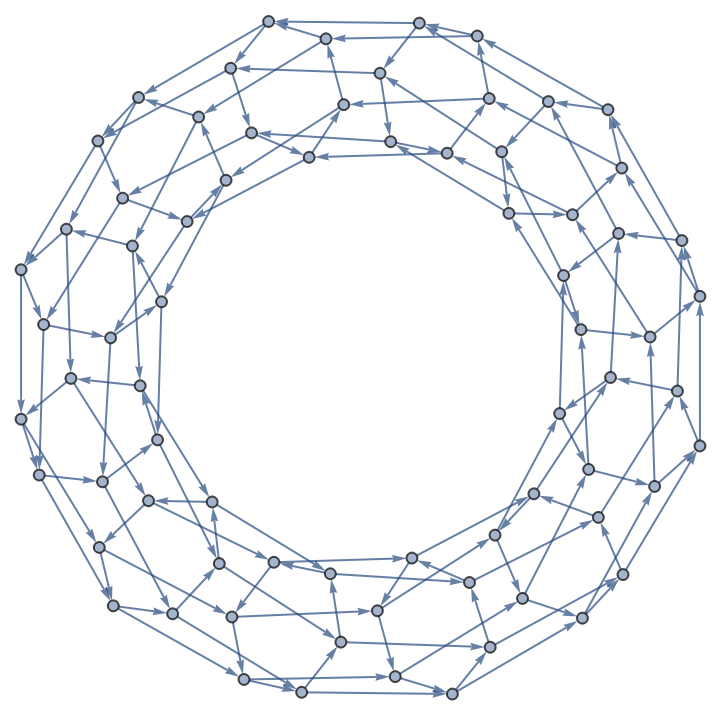Generate a few different Cayley graphs for the Octahedral group:

 In:=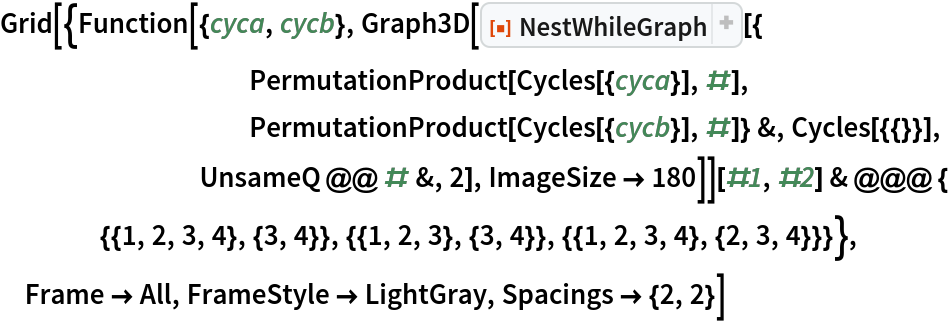Out=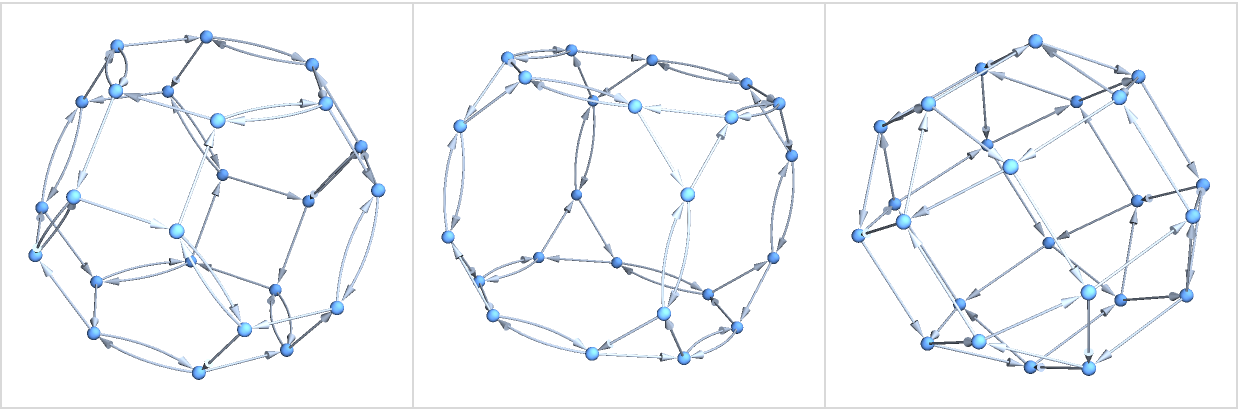### Scope (1)

When setting DirectedEdges to False, outputs become undirected graphs:

 In:=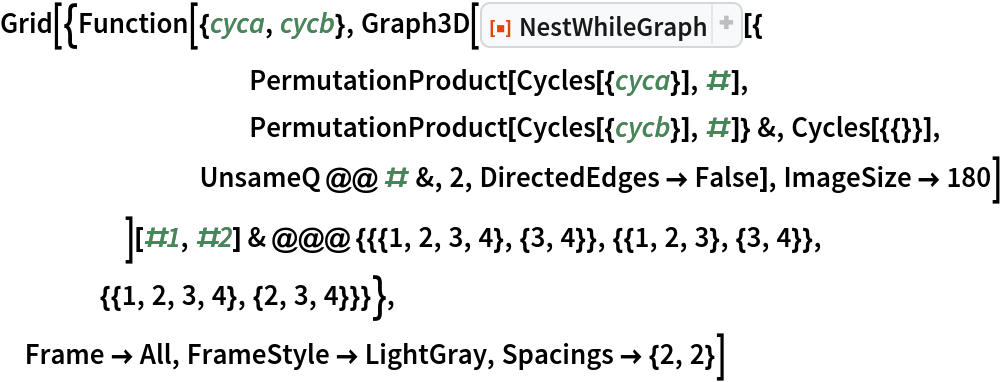Out=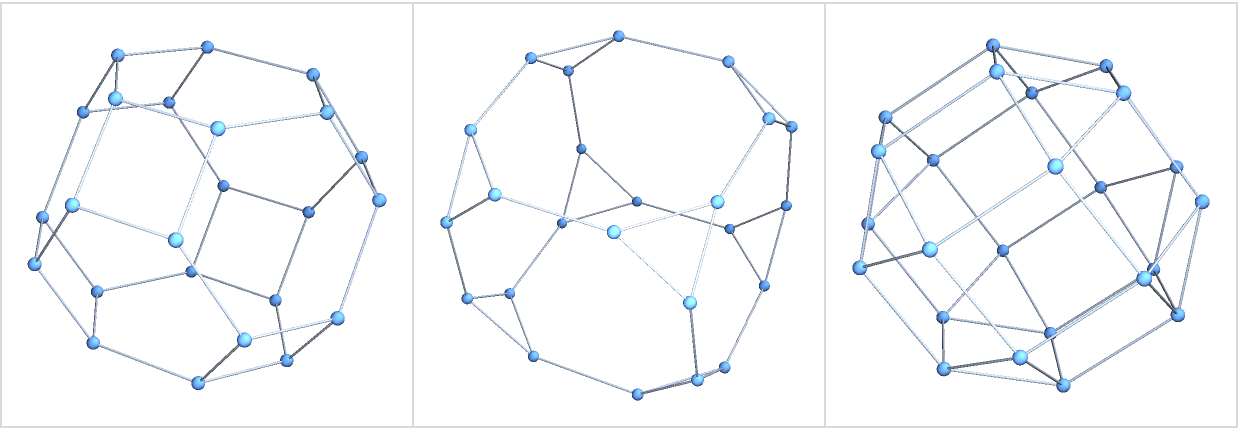### Options (1)

Termination can also be accomplished by introducing a cutoff for recursion depth:

 In:=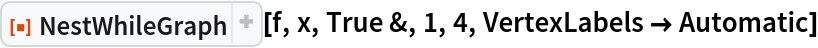Out=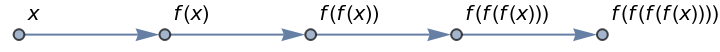### Neat Examples (2)

Construct the game graph of a loopy game:

 In:=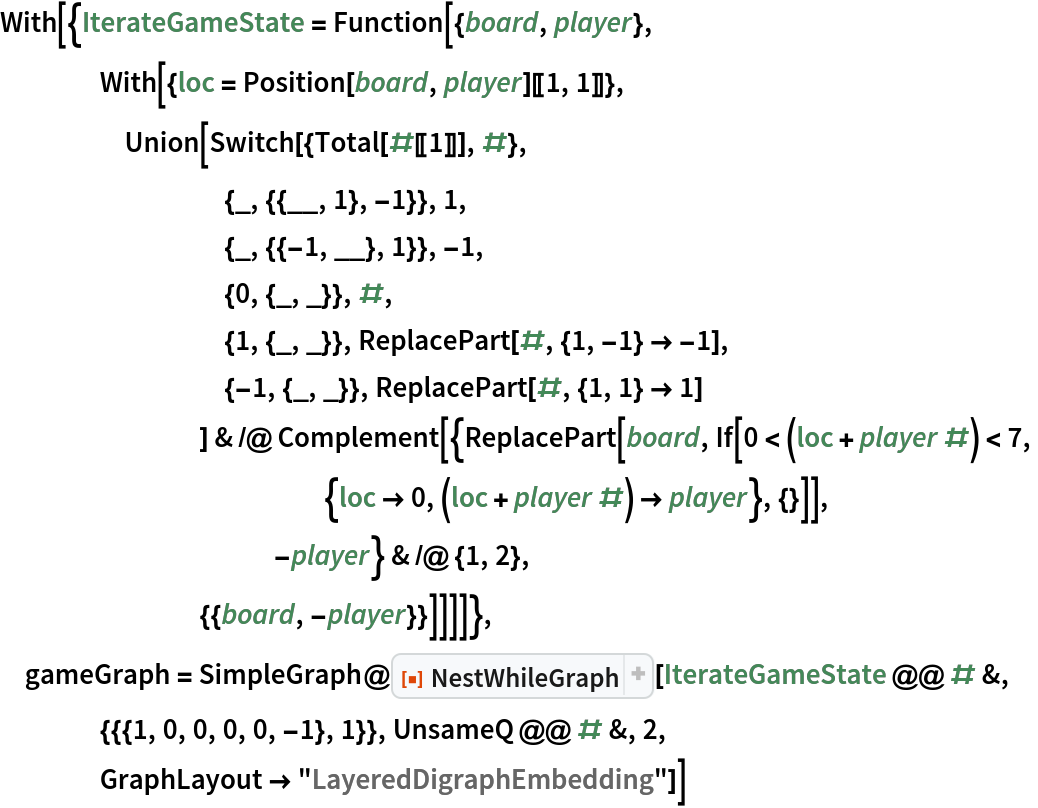Out=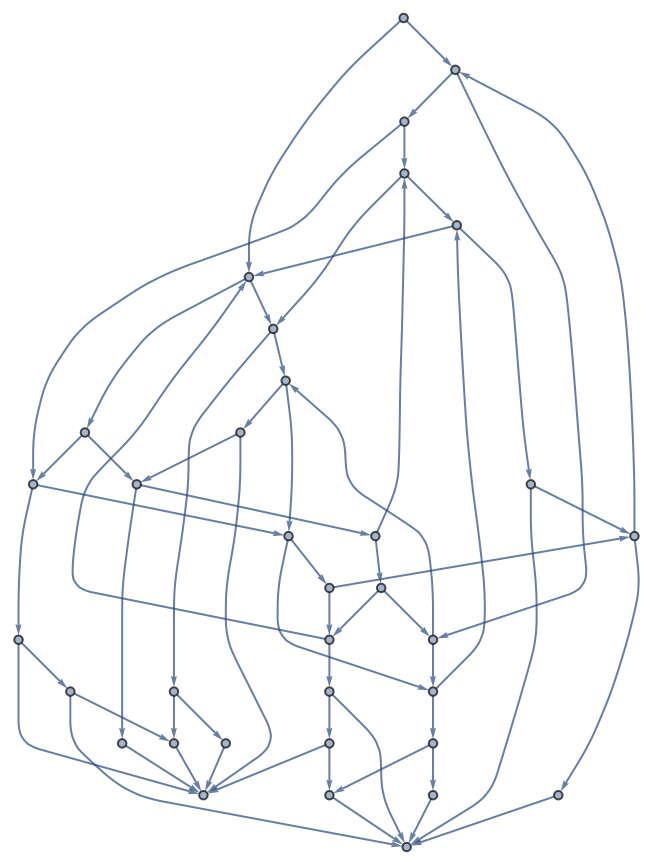Find loops in the game graph:

 In:=Out=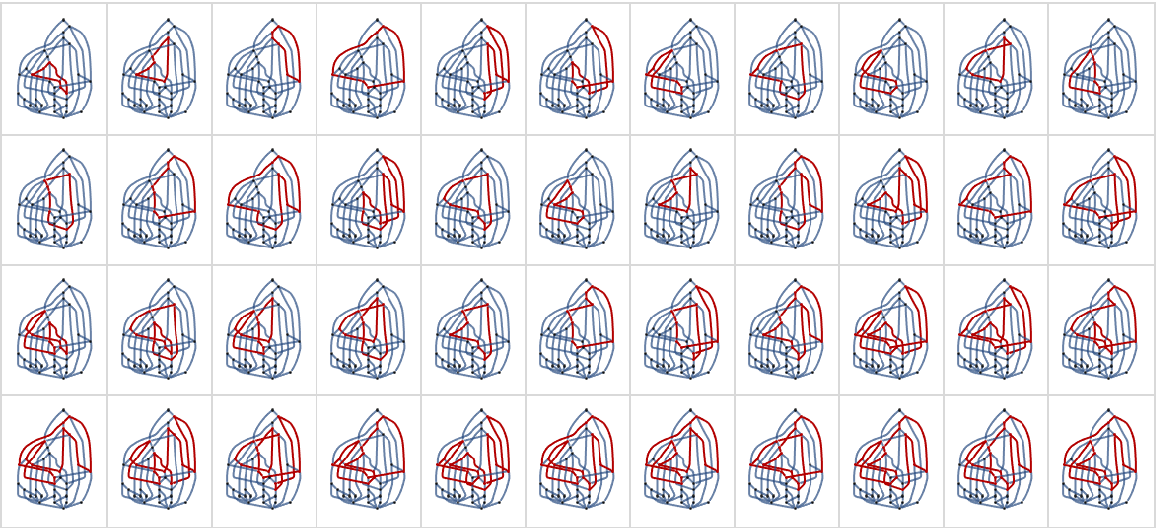Generate a graph with structure related to prime numbers:

 In:=Out=Notice false positives on the growth front:

 In:=Out=